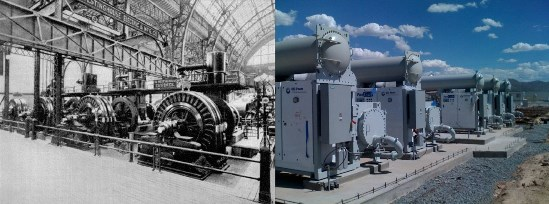As we have seen in the article “Electricity and Magnetism”, moving charges or electricity was producing magnetic fields. The next obvious question to ask would be the contrary. Would magnetic fields or changing magnetic fields produce electricity? Lenz and Faraday almost simultaneously conducted experiments to know whether magnetic fields produced electric current. Lenz’s experiment gave a rather qualitative understanding of the matter. His experiment was simple. He took a current carrying conductor in the form of a loop and connected it to a galvanometer to note any current generated inside the current loop. He moved a bar magnet towards the loop and away from the loop. His observations were also straightforward. The change in the magnetic field did generate current in the loop and the direction of the current was such that it tried to produce a current in the opposite direction so as to oppose the change in the magnetic field inside the loop. Lenz established that the current generated inside the loop always tried to oppose the external magnetic field and hence keeping the conservation of energy intact. We could use a simple thought experiment to prove the conservation of energy. If the current carrying loop were to generate current favoring the direction of the magnetic field, the additional current would further create magnetic field in the same direction as the original. Thus the increase in magnetic field would produce more current and hence further increase in magnetic field can be expected. This process would only lead to an infinite current and an infinite magnetic field, both of which are against any conservation principle. This established that the direction of current as observed by Lenz is in accordance with the law of conservation of energy.

Michael Faraday later conducted a series of experiments where he used a slightly more sophisticated setup using a solenoid and a current carrying loop to establish this time both qualitatively and quantitatively the concept of electromagnetism. He observed the electric current in the current carrying conductor always tried to oppose the rate of change in magnetic flux passing through the loop. This later became one of the famous Maxwell’s equations. Therefore, after a series of experiments, what were once thought to be independent phenomenon (electricity and magnetism) was found to be related. Later James Clerk Maxwell in his 1873 book “A Treatise on Electricity and Magnetism” summarized these interactions and made relativistic corrections to the classical electromagnetic equations. Magnetic flux like electric flux is nothing but the scalar product of the magnetic field intensity and the area vector. Therefore, we have two ways to change the magnetic flux. One of them is to change the angle between the direction of the magnetic field and the area vector which is possible in the case of a rotating loop in the presence of an external magnetic field. This method of changing the magnetic flux to produce electric current carved path to one of the most important technological revolutions of that century. The invention of the AC current. This radically changed how power was to be conceived, generated and transmitted more economically and safely. We have drifted from an older and expensive method of DC power to AC power after a period of turbulence in the power industry. What is called the “War of Currents”, which we discussed briefly in our A Level Physics tuition classes.

Electromagnetic force is now considered one of the four most fundamental forces that behind the functioning of the universe including the Gravitational force, Weak nuclear force and the Strong nuclear force. As the names suggest, the weak and the strong nuclear force operate only under the sub-atomic level. Therefore in all the forces that operate in our daily lives above the atomic level and apart from the gravitational force are all but the electromagnetic forces.